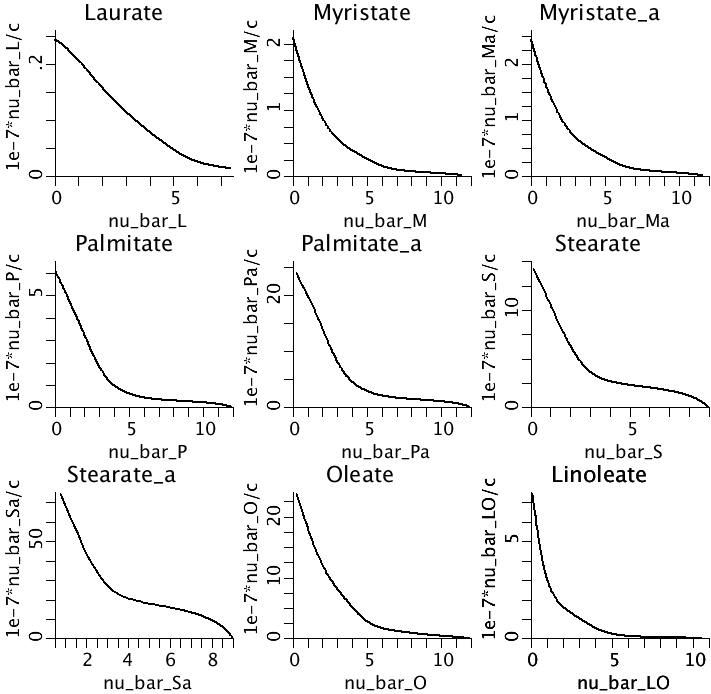# FattyAcidBinding

Model number
0392

Long chain fatty acid binding to human plasma albumin

## Description

```   All constants and intermediate variables have been declared private,
with the exception of the parameters and variables pertaining to
Palmitate (P). Commenting the word private in the model will restore
them to the input and output display on the runtime menu.

The variables nu_bar_XX are the ratios of the average number of moles of
fatty acid bound per mol of albumin.

The independent variable in this model is c, the molar concentration of
the unbound fatty acid in the aqueous phase.

The suffix a indicates that the equilibrium constants were calculated
using corrections for unbound fatty acid association in the albumin
solution.

the nu_bar functions are suffixed L(Laureate), M(Myristate), Ma(Myristate_a),
P(Palmitate) Pa(Palmitate_a), S(Stearate), Sa(Stearate_a), O(Oleate), and
LO(Linoleate).

Calculate
nu_bar =
(
K1 * c
+ 2 * K1 * c^2  * K2
+ 3 * K1 * c^3  * K2 * K3
+ 4 * K1 * c^4  * K2 * K3 * K4
+ 5 * K1 * c^5  * K2 * K3 * K4 * K5
+ 6 * K1 * c^6  * K2 * K3 * K4 * K5 * K6
+ 7 * K1 * c^7  * K2 * K3 * K4 * K5 * K6 * K7
+ 8 * K1 * c^8  * K2 * K3 * K4 * K5 * K6 * K7 * K8
+ 9 * K1 * c^9  * K2 * K3 * K4 * K5 * K6 * K7 * K8 * K9
+ 10 * K1 * c^10 * K2 * K3 * K4 * K5 * K6 * K7 * K8 * K9 * K10
+ 11 * K1 * c^11 * K2 * K3 * K4 * K5 * K6 * K7 * K8 * K9 * K10 * K11
+ 12 * K1 * c^12 * K2 * K3 * K4 * K5 * K6 * K7 * K8 * K9 * K10 * K11 * K12
)
/
(
1
+ K1 * c
+ K1 * c ^ 2 * K2
+ K1 * c ^ 3 * K2 * K3
+ K1 * c ^ 4 * K2 * K3 * K4
+ K1 * c ^ 5 * K2 * K3 * K4 * K5
+ K1 * c ^ 6 * K2 * K3 * K4 * K5 * K6
+ K1 * c ^ 7 * K2 * K3 * K4 * K5 * K6 * K7
+ K1 * c ^ 8 * K2 * K3 * K4 * K5 * K6 * K7 * K8
+ K1 * c ^ 9 * K2 * K3 * K4 * K5 * K6 * K7 * K8 * K9
+ K1 * c ^ 10 * K2 * K3 * K4 * K5 * K6 * K7 * K8 * K9 * K10
+ K1 * c ^ 11 * K2 * K3 * K4 * K5 * K6 * K7 * K8 * K9 * K10 * K11
+ K1 * c ^ 12 * K2 * K3 * K4 * K5 * K6 * K7 * K8 * K9 * K10 * K11 * K12
)

The calculation is shortened by defining T-variables recursively. See the code to
see how this is done.
```

Binding of fatty acids to human plasma albumin at 37o C:## Equations

None.

The equations for this model may be viewed by running the JSim model applet and clicking on the Source tab at the bottom left of JSim's Run Time graphical user interface. The equations are written in JSim's Mathematical Modeling Language (MML). See the Introduction to MML and the MML Reference Manual. Additional documentation for MML can be found by using the search option at the Physiome home page.

Key terms
albumin
laurate
myristate
palmitate
stearate
oleate
linoleate
binding
fatty acid
stepwise equilibrium
Acknowledgements

Please cite https://www.imagwiki.nibib.nih.gov/physiome in any publication for which this software is used and send one reprint to the address given below:
The National Simulation Resource, Director J. B. Bassingthwaighte, Department of Bioengineering, University of Washington, Seattle WA 98195-5061.

Model development and archiving support at https://www.imagwiki.nibib.nih.gov/physiome provided by the following grants: NIH U01HL122199 Analyzing the Cardiac Power Grid, 09/15/2015 - 05/31/2020, NIH/NIBIB BE08407 Software Integration, JSim and SBW 6/1/09-5/31/13; NIH/NHLBI T15 HL88516-01 Modeling for Heart, Lung and Blood: From Cell to Organ, 4/1/07-3/31/11; NSF BES-0506477 Adaptive Multi-Scale Model Simulation, 8/15/05-7/31/08; NIH/NHLBI R01 HL073598 Core 3: 3D Imaging and Computer Modeling of the Respiratory Tract, 9/1/04-8/31/09; as well as prior support from NIH/NCRR P41 RR01243 Simulation Resource in Circulatory Mass Transport and Exchange, 12/1/1980-11/30/01 and NIH/NIBIB R01 EB001973 JSim: A Simulation Analysis Platform, 3/1/02-2/28/07.